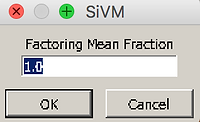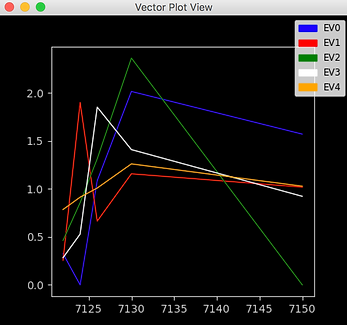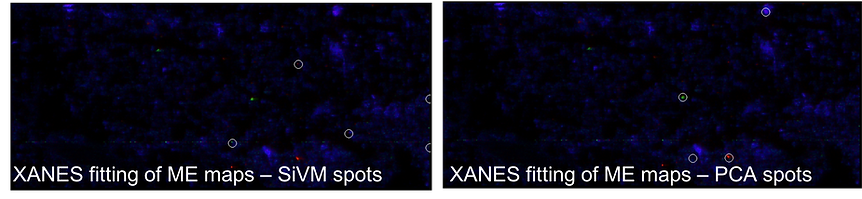top of page

# Non-Negative Matrix Factorization & Simplex Volume Maximization

Top

Non-negative Matrix Factorization (NMF)

PCA is a common and robust way to examine variation in a data set, while minimizing redundancy (i.e. components that have overlapping information) due to the orthogonality constraint. However, the components can be hard to interpret because they do not have any chemical meaning; they simply map the data onto a new set of vectors that are aligned with the direction of greatest variance in the data.

To obtain components that do hold chemical meaning, we can apply NMF instead. NMF is a matrix factorization problem in which the goal is to come up with a set of components that can be thought of as chemical endmembers.

This problem can be expressed as:

X = WH

X is the data matrix, which has dimensions m x n. m represents different types of measurements. In our case, these are intensities of different wavelengths that we measure (in SMAK, this is the number of channels you select). n is the number of measurements; in our case, this is the number of pixels in the map.

W is a matrix of dimensions m x k. W comprises a set of basis vectors that describe the loading of the data set’s variables onto k bases.

H is a matrix of k x n dimensions. The elements of this matrix represent the loading of each k base onto n.

W and H are constrained to be non-negative, hence the term “NMF”

X can be written, column by column, as:

x = Wh

That is to say, that each data vector x can be expressed as a linear combination of the columns of W weighted by the components of h.

Importantly, since k < m, WH only represents a good approximation of X if the basis vectors of W represent the most important chemical constituents of X, that is, if they serve as appropriate chemical endmembers.

NMF

Simplex Volume Maximization (SiVM)

SiVM employs the same relationship as NMF (where W and H are non-negative):

X       = W       H

However, SiVM relies on a geometric solution to obtain W and H, which greatly reduces the computational expense. In geometry, a simplex is a polyhedral shape with k vertices. For instance, a triangle is a simplex with k = 3. In our case, k is the number of bases in W. SiVM seeks to find a set of bases (k) of W that are actual data points. The k data points are chosen such that they maximize the number of data points the simplex encompasses; each k data point thus represents an endmember (or “archetype”) of the whole data set. The coefficients of the matrix H give a measure of the similarity of each element in X to the corresponding archetypes in W.

The following image shows how a simplex is constructed with k = 2, 3 or 4.

Note how the points are chosen to span the largest amount of the data possible.

SiVM clusters data in a very intuitive way: it selects endmembers and then expresses all other points in the data set as a combination of these endmembers. This is the utility and power of SiVM (and NMF, too).

The draw back in using SiVM is that there is a trade-off between adequately describing all data points in the data set and choosing redundant endmembers. If too few endmembers are chosen (i.e., too low a value of k is chosen), then not all points in the data set will be adequately described (i.e. not all data points will lie within the simplex). In the figure above it can be seen that k = 2 and k = 3 are not adequate to describe the majority of the data set. However, as more endmembers are chosen, the endmembers will start to share characteristics; they will not signify unique chemical species. In any case, the choice of k matters for the analysis and it is wise to attempt the same SiVM calculation with a few different values of k.Romer et al., Functional Plant Biology, 2012, 39, 878–890

m x n

m x k

k x n

SiVM

NMF Example

The following example illustrates how NMF can be used to identify distinct regions in a sample that exhibit different chemical forms of Fe. The sample consists of a biofilm of Fe(II)-oxidizing bacteria, which had been collected from a pond, that was drop deposited on a plastic microscope slide. Fe(II)-oxidizing bacteria produce Fe(III) oxy(hydr)oxides. In this example, the researcher was curious to know whether all of the Fe present in the biofilm was Fe(III), or if some Fe(II) was present. Maps were collected at 7122 eV, 7124 eV, 7126 eV, 7130 eV, and 7150 eV.

NMF (and SiVM) is accessed through the PCA button on the main SMAK window:

Select the channels on which the NMF will be performed, then select NMF. New channels will then appear in the SMAK data channels list ("NMFcomp1", etc). Since we started with five channels (five different energies), we will generate 5 NMF components.

The NMF components are shown here:

Additionally, the following plot will be generated which shows the NMF endmember vectors (in order to make sure the x-axis is a function of the selected energies, follow instructions here                                               ):

Unlike PCA, NMF vectors are not orthogonal to one another. Thus, there is more similarity among the NMF component images than among the                       . NMF component 1 is likely to represent concentration gradients (as is also true for PCA). In this particular example, NMF Component 2 appears the most different from the other components: it exhibits a small number of bright spots, whereas NMF components 3 and 4 appear to have a very similar intensity distribution as NMF component 1. We therefore infer that NMF components 3 and 4 may not contain chemical forms of Fe that are entirely distinct from component 1.  NMF component 5 exhibits the smallest intensity differences across the image, so we can choose to neglect this endmember. To select spots for XANES that we anticipate will be chemically distinct we should therefore prioritize picking bright spots from NMF components 1 and 2.

It can be seen that the features selected via NMF and PCA are very similar (open circles denote locations of XANES spots):NMF imges
SMAK exampleSiVM Example

As for PCA and NMF, SiVM is accessed by selecting the green PCA button on the main SMAK window, selecting the channels of interest (in this example, these are the energies from the multienergy map: 7122, 7124, 7126, 7130, and 7150 eV), and then selecting SiVM:

Two more pop up windows will appear. One will ask what you how many clusters you would like to use. The default selection is equal to the number of channels you are running the SiVM analysis on (in this case 5), but it is wise to try SiVM with a few different values and see how the analysis changes. You can also select the Factoring Mean Fraction. Values less than one will enhance your sensitivity to minor signals in your sample. The default value is 1:

The SiVM components are shown below. Note that the coordinates of the endmembers will automatically be selected for XANES analysis. The "Plot Marker Option" window will automatically pop up with these coordinates listed. The open circles on the component images show where these coordinates are located.

Additionally, the following plot will be generated which shows the SiVM endmember vectors (in order to make sure the x-axis is a function of the selected energies, follow instructions here                                               ):

It is instructive to examine the points selected via PCA (and NMF analysis, which was similar to PCA) and SiVM. The following plot shows the results of                           of the multienergy (ME) maps, with the red and green spots indicating Fe(II)-containing species and the blue spots indicating Fe(III). One can see that is this specific example, PCA (and NMF) did a better job of finding spots than SiVM. The take home message is that it is important to try multiple methods to find XANES locations- PCA, NMF and SiVM.SiVM examplebottom of page